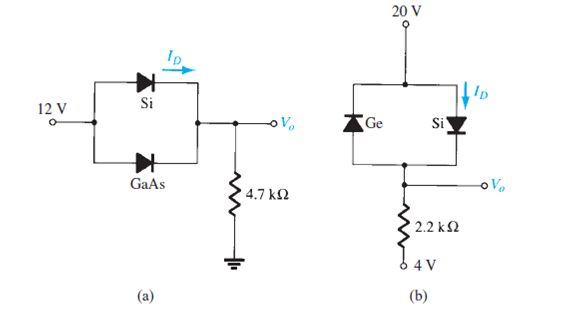Problem

# Determine Vo and ID for the networks of Fig. 2.160.FIG. 2.160

Determine Vo and ID for the networks of Fig. 2.160.

FIG. 2.160#### Step-by-Step Solution

Solution 1

(b)

Refer to Figure $$2.160$$ (b) from the textbook.

Assume silicon diode is forward bias and Germanium diode is reverse biased.

Check the correctness of the assumption. If the current flow through the Si diode is positive, then the assumption is correct.

\begin{aligned} I_{D} &=\frac{20 \mathrm{~V}-4 \mathrm{~V}-0.7 \mathrm{~V}}{2.2 \mathrm{k} \Omega} \\ &=6.95 \mathrm{~mA} \end{aligned}

Current flow through the Si diode is positive quantity. Hence, the assumption is correct.

Therefore, the diode current $$I_{D}$$ is $$6.95 \mathrm{~mA}$$.

Calculate the output voltage $$V_{o}$$.

\begin{aligned} V_{o} &=20 \mathrm{~V}-0.7 \mathrm{~V} \\ &=19.3 \mathrm{~V} \end{aligned}

Hence, output voltage $$V_{o}$$ is $$19.3 \mathrm{~V}$$.

(a)

Refer to Figure $$2.160$$ (a) from the textbook.

From the Figure $$2.160$$ (a), both the Silicon (Si) and Gallium Arsenide (GaAs) diodes are forward based. Silicon diode offset voltage is $$0.7 \mathrm{~V}$$ and GaAs diode offset voltage is $$1.2 \mathrm{~V}$$.

Increasing supply voltage reaches $$0.7 \mathrm{~V}$$ first. The silicon diode will turn "on" and maintained the level of $$0.7 \mathrm{~V}$$. The result is that the voltage across Gallium Arsenide (GaAs) diode will never rise above $$0.7 \mathrm{~V}$$ and remains acts as open circuit.

Hence, the Silicon diode turns on first and gives $$0.7 \mathrm{~V}$$ drop. Silicon diode "on" preventing GaAs diode from turning on.

Find current flow through resistor $$I_{R}$$.

\begin{aligned} I_{R} &=\frac{12 \mathrm{~V}-0.7 \mathrm{~V}}{4.7 \mathrm{k} \Omega} \\ &=2.4 \mathrm{~mA} \end{aligned}

The same current flow through the diode.

\begin{aligned} I_{R} &=I_{D} \\ &=2.4 \mathrm{~mA} \end{aligned}

Therefore, current flow through the diode $$I_{D}$$ is $$2.4 \mathrm{~mA}$$.

Silicon diode voltage $$V_{D}$$ is $$0.7 \mathrm{~V}$$

Calculate the output voltage $$V_{p}$$.

\begin{aligned} V_{o} &=12 \mathrm{~V}-0.7 \mathrm{~V} \\ &=11.3 \mathrm{~V} \end{aligned}

Therefore, output voltage $$V_{o}$$ is $$11.3 \mathrm{~V}$$.